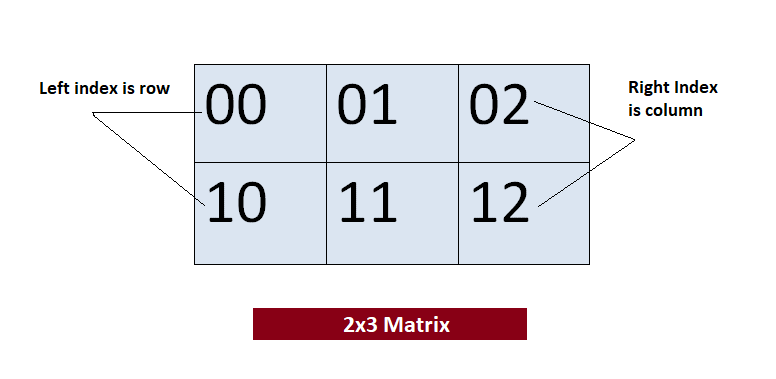## 05 Feb C# Multi-Dimensional Arrays

In the last lesson we learned about One-Dimensional Arrays in Java and how to use them. Now, we will see another type of arrays in C# i.e. Multidimensional Arrays. Multi-dimensional arrays as the name suggests are arrays of arrays.  These are also called rectangular arrays. Multi-Dimensional can be the following in C#:

• Two-Dimensional Arrays
• Three-Dimensional Arrays

## Declare and Initialize a Multi-Dimensional Array

To create a Multi-Dimensional array, set a comma inside the square bracket. You need to use two square brackets to declare a multidimensional array.

For a Two-Dimensional array, set a single comma as shown below:

We can also directly declare and initialize a Two-Dimensional array:

For a Three-Dimensional array, set two comma’s as shown below:

The following figure displays how a multidimensional array forms a 2×3 matrix,In the above figure, you can see the left index determines row and the right index determines column.

## Example – Multi-Dimensional Arrays in C#

Let us now see an example to display each element from our 2×3 matrix,

Output

## Example – Access elements of a Multi-Dimensional Array in C#

We can easily access the elements of a two-dimensional array. Set the array name and then two indexes i.e.

Let us follow what we saw above in the following example:

Output[email protected]

We work to create programming tutorials for all.